### Fixed crack model for fiber reinforced composites - FRCFCM

This material model is an extension of the ConcreteFCM described in Section 1.6.11.

It is possible to choose from three different “classes” of fibers. This choice is controlled by keyword fiberType: 0 = continuous aligned fibers (CAF), 1 = short aligned fibers (SAF) and 2 = short random fibers (SRF). Currently, it is not possible to combine more classes of fibers in one material model.

All of the above-mentioned fiber types are further defined by the material properties and geometry. Fiber quantity is captured by the dimensionless volume fraction Vf (as decimal). All fibers are assumed to have a circular cross-section (shape factor for shear kfib with default value 0.9) and to possess the same geometry characterized by the diameter Df and length Lf.

The overall elastic stiffness of the fiber-reinforced composite is calculated as a weighted average of the moduli of matrix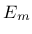and fibers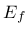and the Poisson's ratio is considered to be equal to the Poisson's ratio of the matrix. Similarly to ConcreteFCM, cracking is initiated once the tensile stress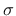in matrix reaches the tensile strength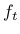.

The nominal bridging stress for continuous aligned fibers which are not perpendicular to the crack plane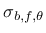can be very easily obtained by multiplying the nominal bridging stress for perpendicular fibers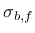by two terms: the first one reflecting lower volume of inclined fibers passing through the crack plane and the second one capturing the snubbing effect: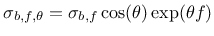(179)

where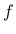is a snubbing coefficient.

For the CAF perpendicular to the crack, the nominal bridging stress can be derived as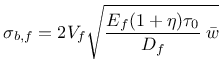(180)

where.

For SRF the nominal bridging stress is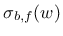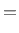(181)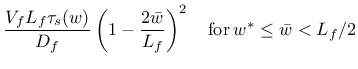(182)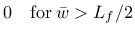(183)

where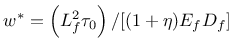;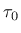is the bond shear strength between the fiber and matrix for small crack openings,.

Larger pull-out displacements can lead to significant physical changes in the fiber surface which can result into changes in the bond shear stress. This phenomenon is captured by function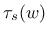relating the frictional bond to the crack opening and is implemented in three alternative formulations. (In order to keep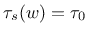use fssType = 0.) In conventional FRC with ordinary concrete matrix, the frictional bond usually decreases with increasing slip. To capture this type of behavior we adopt the function proposed by Sajdlová (activated with fssType = 1) reads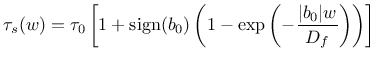(184)

where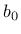is a micromechanical parameter. In composites with high-strength matrix and coated high-strength steel fibers (HSFRC, UHPFRC) as well as in SHCC materials with polymeric fibers, the frictional bond-slip relation often exhibits hardening; this phenomenon can be well approximated by a cubic function (activated with fssType = 2) proposed by Kabele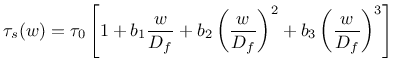(185)

or an alternative formulation which results in smooth changes in the bridging stress (activated with fssType = 3)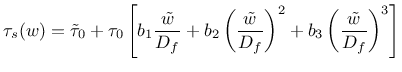(186)

In the last two equations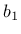,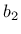and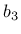are micromechanical parameters and additionally in the last equation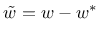,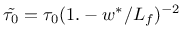for SRF and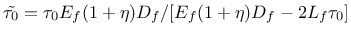for SAF.

The bridging stress for SRF is defined as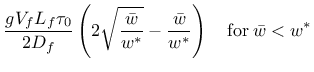(187)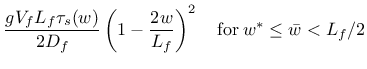(188)(189)

where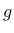is the snubbing factor is defined as(190)

It is possible to delay the activation of the stress in fibers using prameteter fibreActivationOpening which can be imagined as a “lag” of the fiber-related crack opening behind the matrix-related crack opening.

During unloading the stress in fibers does not decrease linearly to origin. Current implementation uses a power function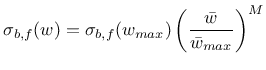(191)

where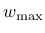is the maximum crack width reached in the entire previous history and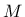is a positive constant, its default value is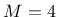.

The influence of crack opening and sliding on the bridging shear stress only due to fibers is expressed as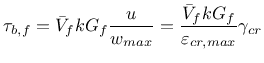(192)

where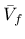is the effective volume of fibers crossing a crack plane (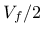for SRF andfor CAF and SAF), and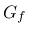is the fiber shear modulus. This expression is motivated by assumption that the fibers bridging the crack planes behave as the Timoshenko beams subjected to shear. Note that the shear stiffness of fibers is not recovered upon unloading.

It has been found that in some high performance fiber reinforced cement composites, fibers rupture when cracks are exposed to shearing. This phenomenon is modeled by damage parameter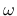, which accounts for the ratio of ruptured fibers and varies between the values of 0 and 1. It is assumed thatdepends on the maximum shear strain sustained by the protruding portions of bridging fibers throughout the loading history. This crack shear strain can be expressed as: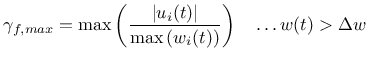(193)

whereis the crack sliding displacement (CSD) and and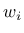is the maximum value of the crack opening displacement of the i-th crack. This means that the damage does not grow if the crack closes (crack opening decreases). If more cracks exist, the maximum contribution is considered.

Two different one-parameter damage evolution laws are currently implemented. For fDamType = 0 the damage is deactivated, with fDamType = 1 damage is described by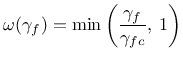(194)

and finally with fDamType = 2(195)

where(gammaCrack in the input record) is a parameter.

Since damage reduces the number of crack-bridging fibers, which is proportional to the fiber volume fraction, its effect can be suitably implemented by introducing the effective volume fraction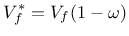(196)

The material parameters are summarized in Tables 42 (matrix) and 43 (fiber extension).

Sample syntax for a fixed crack model reinforced with fibers with volume density 24 kN/m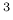, thermal dilation coefficient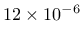K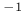, Young's modulus of the matrix 20 GPa, Poisson's ratio of matrix 0.2, fracture energy of matrix 100 N/m, tensile strength of matrix 2 MPa, linear tension softening, constant shear retention factor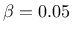, unlimited shear strength (shearStrengthType = 0), continuous aligned fibers, fiber volume 2%, fiber diameter 0.04 mm, Young's modulus of fibers 20 GPa, shear modulus of fibers 1 GPa, fiber-matrix bond strength 1 MPa, snubbing coefficient 0.7, shear correction coefficient 0.9, deactivated fiber damage, fiber act if COD exceeds 10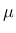m (with smoothing from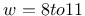m), fiber orientation at 45 degrees in x-y plane, automatic evaluation of crack spacing from composition; the analysis uses [m], [MPa] and [MN]:
FRCFCM 1 d 24.e-3 talpha 12.e-6 E 20000. n 0.2 Gf 100e-6 ft 2.0
softType 2 shearType 1 beta 0.05 FiberType 0 Vf 0.02 Df 0.04e-3
Ef 20000. Gfib 1000. tau_0 1. FSStype 0 f 0.7 kfib 0.9 fDamType 0
fibreactivationopening 10.e-6 dw0 2.e-6 dw1 1.e-6 orientationVector 3 1. 1. 0. computeCrackSpacing

Table 43: Fixed crack model for fiber reinforced concrete - summary.
Description Fixed crack model for FRC
Record Format FRCFCM input record of ConcreteFCM Vf(rn) # Lf(rn) # Df(rn) # Ef(rn) # [ nuf(rn) #] [ Gfib(rn) #] [ kfib(rn) #] tau_0(rn) # b0(rn) # b1(rn) # b2(rn) # b3(rn) # f(rn) # [ M(in) #] [ fibreOrientationVector(ra) #] [ fssType(in) #] [ fDamType(in) #] [ fiberType(in) #] [ gammaCrack(rn) #] [ computeCrackSpacing ] [ fibreActivationOpening(rn) #] [ dw0(rn) #] [ dw1(rn) #]
Parameters - Vf fiber content expressed as decimal
- Lf fiber length
- Df fiber diameter
- Ef fiber Young's modulus
- nuf fiber Poisson's ratio
- Gfib fiber shear modulus (read when nuf is not provided)
- kfib fiber cross-sectional shape correction factor
- tau_0 bond shear strength at zero slip
- b0 micromechanical parameter for fiber shear according to Sajdlová
- b1, b2, b3 micromechanical parameter for fiber shear according to Kabele
- f snubbing friction coefficient
- M exponent related to fiber unloading
- fibreOrientationVector vector specifying orientation for CAF and SAF fibers
- fssType type of Fiber bond Shear Strength (bond shear strength vs. crack opening)
 0 - constant shear strength 1 - bond shear strength with parameter b0 2 - bond shear strength with parameters b1, b2, b3 3 - bond shear strength with parameters b1, b2, b3 which leads to smooth traction-separation law
- fDamType type of damage law for fibers
 0 - no damage 1 - damage controlled by shear slip deformation of the crack (with gammaCrack), linear law 2 - damage controlled by shear slip deformation of the crack (with gammaCrack), exponential law
- fiberType type of reinforcing fibers
 0 - CAF (continuous aligned fibers) 1 - SAF (short aligned fibers) 2 - SRF (short randomly oriented fibers)
- gammaCrack crack shear strain parameter applicable with fDamType = 1 or 2 (here the crack shear strain is understood as the crack slip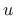divided by the crack opening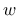)
- computeCrackSpacing crack spacing is evaluated automatically based on provided composition
- fibreActivationOpening crack opening at which the fibers begin transferring bridging stress
- dw0, dw1 applicable only if fibreActivationOpening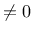, then it allows to smooth the traction-separation law for fibers; lower bound is fibreActivationOpening - dw0 and the upper bound is fibreActivationOpening - dw1
Supported modes 3dMat, PlaneStress, PlaneStrain

Borek Patzak
2019-03-19